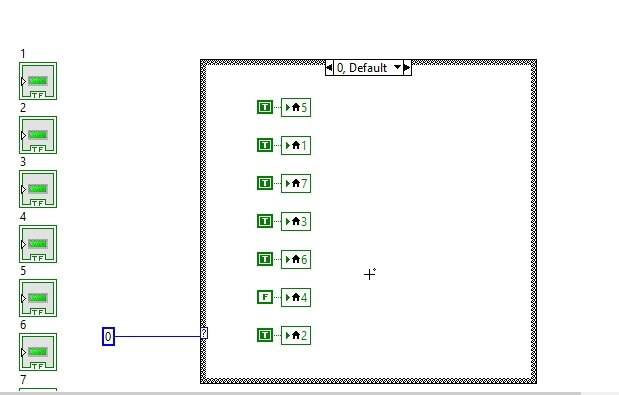# Seven segment display in labview : tutorial 25

In this tutorial I will help you to design a VI that will display the number of your demand on a seven segment LED display. You will provide a constant value as input and at VI will display your entered constant on a seven segment display. Using all the knowledge you have gained so far, you will be designing a simple VI as a sample project in this tutorial. At the end of the tutorial you are provided with an exercise to do it by yourself, and in the next tutorials I will assume that you have done those exercises and I will not explain the concept regarding them.

### Seven segment display in labview  example

Figure 1: Square LED

Figure 2: Seven segment arrangement

Figure 3: Case structure placement

Figure 4: Constant placement

Figure 5: Numeric condition

Figure 6: Local variables

Figure 7: Case 0 of case structure

Figure 8: Case 1 of case structures

Figure 10: Creating constant

Figure 11: Constants creation in case 0

Figure 12: Constants creation in case 1

• Do the same with all the 10 cases of LEDs you created before.
• Now from the seven segments arrangement you designed earlier in this tutorial, draw a pattern of LEDs which needs to be turn on in case 0. i.e. for case 0, the input will be 0 and the seven segment must display 0 on the LEDs. To do so, all LEDs except 4 in the display should be turned ON. Hence except 4 make all the constants on the remaining LEDs as true, as shown in the figure below,Figure 13: Case 0 LED pattern

Figure 14: Case 1 LED pattern

Figure 15: Case 2 LED pattern

Figure 16: Output with 9 as input

Figure 17: Output with 7 as input

Exercise:

• Run a loop and display all the digits from 0 to 9 on the seven segment with a time difference of 1 sec between two consecutive digits, and at the end of the loop display a loop completion message.

<< Previous tutorial                                      Next tutorial>>